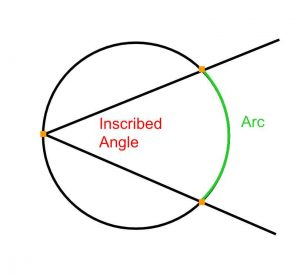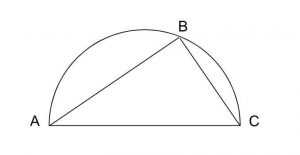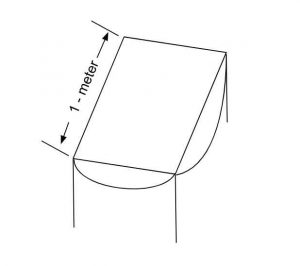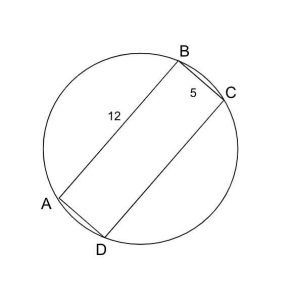Welcome back to our third article on GMAT circles. In the second article, we explored central angles, sectors, and arcs. This time we will introduce another kind of angle: the inscribed angle.

An inscribed angle is an angle drawn by using line segments to connect one point on a circle to two other points on the same circle, as in the graphic below:Like a central angle, an inscribed angle creates a “wedge” shape, like a triangle where one side is rounded. The rounded side is an arc of the circle. For a central angle, the measure of the angle corresponds to the measure of the associated arc in a 1:1 relationship. For an inscribed angle, the measure of the angle corresponds to the measure of the associated arc in a 1:2 relationship. A 30 degree inscribed angle creates a 60-degree arc on the other side of the circle. A 60-degree inscribed angle creates a 12- degree arc on the other side of the circle. And, importantly, a 90-degree inscribed angle creates a 180-degree arc (half a circle or a semicircle) on the other side of the circle.

## 1. Inscribed Angle – GMAT Official Guide Problem

GMAT problems rarely use the term “inscribed angle” or feature an inscribed angle in isolation. Usually, the inscribed angle is part of an inscribed polygon, a polygon drawn inside a circle so that its vertices are points on the circle. Take a look at this official GMAT problem:In the figure shown, the triangle is inscribed in the semicircle. If the length of line segment AB is 8 and the length of line segment BC is 6, what is the length of arc ABC?

A. 15π
B. 12π
C. 10π
D. 7π
E. 5π

The problem refers not to an angle inscribed in a circle but to a triangle inscribed in a semicircle. Still, knowing the “1:2” factor of relationship between an inscribed angle and its associated arc is the key to solving this problem. Your logic might go something like this:

1. This is a semicircle or a 180-degree arc.
2. The angle at point B “opens up” to the straight edge of the semicircle, which is like the diameter of a circle. Another semicircle or 180-degree arc could be drawn across from this angle so that it makes a whole circle with the existing piece.
3. Since the measure of an inscribed angle is 1/2 the measure of the arc it “creates” on the other side of the circle, the angle at point B is a 90-degree angle, and the triangle is a right triangle.

At this point, your attention should return to the given information about the lengths of line segments AB and BC, which we now know to be the legs of a right triangle. These legs have lengths 6 and 8, which have a 3:4 relationship. Therefore we are looking at a 3-4-5 triangle, and the length of the hypotenuse is 10.

Finally, you must recall that this hypotenuse is the diameter of the circle. Therefore the diameter of the whole circle is 10. However, marking answer choice C would be a mistake, since we were asked for the length of arc ABC, where arc ABC is a semicircle (half a circle). So your final step is to divide your diameter of 10π by 2, leading you to the correct answer choice: E.

## 2. Inscribed Square – GMAT Official Guide Problem

Let’s try another problem, this time with an inscribed square:The figure shows a drop-leaf. With all four leaves down the tabletop is a square, and with all four leaves up the tabletop is a circle. What is the radius, in meters, of the tabletop when all four leaves are up?
A. 1/2
B.
√2/2
C. 1
D. √2
E. 2

Notice that the problem doesn’t mention “a square inscribed in a circle,” but that is nonetheless what we have here. Many GMAT quant problems create scenarios that correspond to some mathematical phenomenon without using the math language. In this case, the fact that we are dealing with a square inscribed in a circle is relatively easy to see.

As in the previous problem, we are asked for a value of the circle (this time it is the radius instead of an arc length) but given only information about the inscribed shape: a square. As in the previous problem, the key is realizing that with any 90-degree inscribed angle, the line segments forming the angle are legs of a right triangle whose hypotenuse is also a diameter of the circle.

Using the Pythagorean theorem, the hypotenuse of this triangle (or the diagonal of the inscribed square) is √2. As before, forgetting to divide this value by 2 (since we were asked for the radius, not the diameter) will lead you to an incorrect answer choice. Don’t trip at the finish line. The value you need is √2 /2, answer choice B.

Here is a related problem:If rectangle ABCD is inscribed in the circle above, what is the area of the circular region?

A. 36.00
B. 42.25
C. 64.00
D. 84.50
E. 169.00

Again, we are asked for a value of the circle (its total area) but given only information about the inscribed rectangle. For our purposes, this rectangle is just as good as the square in the previous problem. With the square, we only needed the length of one side, because we know that all four sides are the same length. With a rectangle, we need both the length and the width in order to calculate the diagonal – the diameter of the circle – via the Pythagorean theorem. If you know your Pythagorean triples (like 3-4-5), you may realize immediately that the diagonal of this rectangle is 13.

D = √(5² + 12²)
D = √(25 + 144)
D = √169
D = 13

Now that we have the circle’s diameter, we can solve for its area. The radius of the circle is 13/2 or 6.5, and since Area = r², the square of 13/2 or 6.5 will be the coefficient of in the correct answer choice. It would be a waste of time to fully multiply out 6.5 * 6.5. We know that it will be of form __.25, and the only answer choice that matches this is B.

## 3. Data Sufficiency – GMAT Official Guide Problem

Let’s transition to data sufficiency for one final problem. Using the diagonal/diameter relationship in the previous problems, it would be possible to construct a variety of DS problems. But some DS inscription problems rely on another property of inscribed polygons.

Square ABCD is inscribed in circle O. What is the area of square region ABCD?

1. The area of circular region O is 64π.
2. The circumference of circle O  is 16π.

### Solution

To answer this problem, all you need to know is that there is only one way to inscribe a square in a circle. The vertices of the square must lie on the circle. The perimeters and areas of the square and the circle will scale together. This means that if we know any value for either shape, we can calculate every value for both of them. Therefore each statement on its own is sufficient, and the answer to this problem is D.

As long as any polygon can be established as regular (having sides of equal length and angles of equal measure), there is only one way to inscribe it in a circle. A square is a regular quadrilateral, so this works for squares every time. But this same problem could have used a regular pentagon, a regular hexagon, or any regular polygon you like, and the correct answer would still be D. The regularity of the polygon is sufficient – and necessary – for this to work. If the regularity of the polygon cannot be established, then there are an infinite number of ways to inscribe it in a circle, each with its own unique area and perimeter.

It is also possible to flip the relationship and inscribe a circle inside a polygon. A related term is circumscription. The shape on the inside is inscribed in the shape on the outside. The shape on the outside is circumscribed around the shape on the inside. GMAT problems where the circle is on the inside usually use a square, so that the diameter of the circle is equal to the length of each side of the square. Such problems tend to be of lower difficulty level.

This concludes our third article on the GMAT’s treatment of circles. Next time we will look at what happens when the radius – rather than the diameter – pulls double duty as the hypotenuse of a right triangle.

Contributor: Elijah Mize (Apex GMAT Instructor)